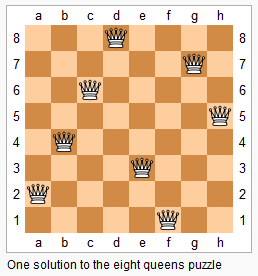## N-Queens

06/27/2016 Hash Table Backtracking

## Question

The n-queens puzzle is the problem of placing n queens on an n×n chessboard such that no two queens attack each other.Given an integer n, return all distinct solutions to the n-queens puzzle.

Each solution contains a distinct board configuration of the n-queens’ placement, where ‘Q’ and ‘.’ both indicate a queen and an empty space respectively.

For example, There exist two distinct solutions to the 4-queens puzzle:

[
[".Q..",  // Solution 1
"...Q",
"Q...",
"..Q."],

["..Q.",  // Solution 2
"Q...",
"...Q",
".Q.."]
]


## Solution

Result: Accepted Time: 4 ms

Here should be some explanations.

class Solution {
public:
void dfs(int row,const int n,vector<vector<string>> & ret,vector<string> & vect,int col[],int xy[],int yx[]){
if(row == n) return ret.push_back(vect);
for(int i = 0; i < n; i++)
if(!col[i] && !xy[n + row - i] && !yx[row + i]){
col[i] = xy[n + row - i] = yx[ row + i] = 1;
vect[row][i] = 'Q';
dfs(row + 1,n,ret,vect,col,xy,yx);
vect[row][i] = '.';
col[i] = xy[n + row - i] = yx[ row + i] = 0;
}
}
vector<vector<string>> solveNQueens(int n) {
int col={0},xy={0},yx={0};
vector<vector<string>> ret;
vector<string> vect(n,string(n,'.'));
dfs(0,n,ret,vect,col,xy,yx);
return ret;
}
};


Complexity Analytics

• Time Complexity: $O(n!)$
• Space Complexity: $O(n*n?)$